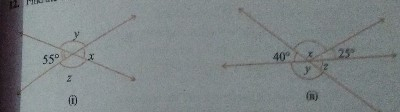"
">

# Find the values of the angles $x, y,$ and $z$ in each of the following:"

To do:

We have to find the values of the angles $x, y,$ and $z$ in the given figures.
Solution:
We know that,

Vertically opposite angles are equal.

Sum of the angles in a linear pair is $180^o$.

Therefore,

(i) $x=55^o$  (Vertically opposite angles)

$y+55^o=180^o$  (Linear pair)

$y=180^o-55^o$

$y=125^o$

$z=y=125^o$  (Vertically opposite angles)

(ii) $z=40^o$  (Vertically opposite angles)

$y+40^o=180^o$  (Linear pair)

$y=180^o-40^o$

$y=140^o$

$z+x+25^o=180^o$  (Linear pair)

$x=180^o-25^o-40^o$

$x=115^o$

Updated on: 10-Oct-2022

25 Views